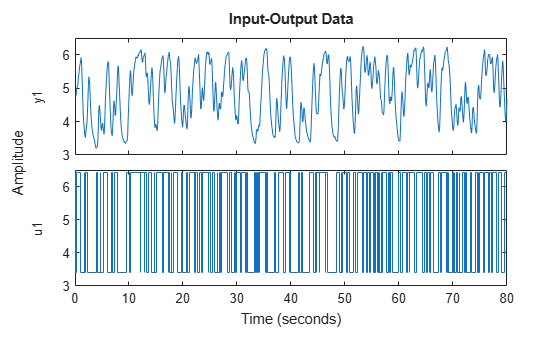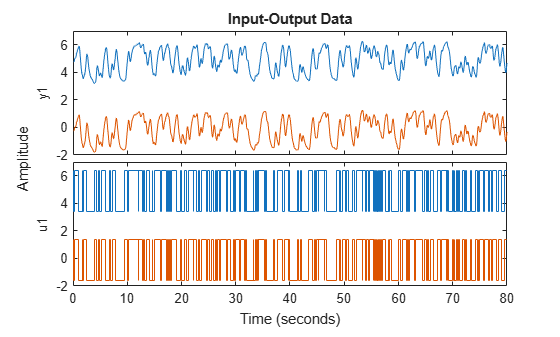Documentation

TrendInfo

Offset and linear trend slope values for detrending data

Description

TrendInfo class represents offset and linear trend information of input and output data. Constructing the corresponding object lets you:

• Compute and store mean values or best-fit linear trends of input and output data signals.

• Define specific offsets and trends to be removed from input-output data.

By storing offset and trend information, you can apply it to multiple data sets.

After estimating a linear model from detrended data, you can simulate the model at original operation conditions by adding the saved trend to the simulated output using retrend.

Construction

For transient data, if you want to define a specific offset or trend to be removed from this data, create the TrendInfo object using getTrend. For example:

T = getTrend(data)

where data is the iddata object from which you will be removing the offset or linear trend, and T is the TrendInfo object. You must then assign specific offset and slope values as properties of this object before passing the object as an argument to detrend.

For steady-state data, if you want to detrend the data and store the trend information, use the detrend command with the output argument for storing trend information.

Properties

After creating the object, you can use get or dot notation to access the object property values.

Property NameDefaultDescription
DataName''Name of the iddata object from which trend information is derived (if any)
InputOffsetzeros(1,nu), where nu is the number of inputs
• For transient data, the physical equilibrium offset you specify for each input signal.

• For steady-state data, the mean of input values. Computed automatically when detrending the data.

• If removing a linear trend from the input-output data, the value of the line at t0, where t0 is the start time.

For multiple experiment data, this is a cell array of size equal to the number of experiments in the data set.

InputSlopezeros(1,nu), where nu is the number of inputs

Slope of linear trend in input data, computed automatically when using the detrend command to remove the linear trend in the data.

For multiple experiment data, this is a cell array of size equal to the number of experiments in the data set.

OutputOffsetzeros(1,ny), where ny is the number of outputs
• For transient data, the physical equilibrium offset you specify for each output signal

• For steady-state data, the mean of output values. Computed automatically when detrending the data.

• If removing a linear trend from the input-output data, the value of the line at t0, where t0 is the start time.

For multiple experiment data, this is a cell array of size equal to the number of experiments in the data set.

OutputSlopezeros(1,ny), where ny is the number of outputs

Slope of linear trend in output data, computed automatically when using the detrend command to remove the linear trend in the data.

For multiple experiment data, this is a cell array of size equal to the number of experiments in the data set.

Examples

collapse all

Remove specified offset from input and output signals.

Load SISO data containing vectors u2 and y2.

Create a data object with sample time of 0.08 seconds and plot it.

data = iddata(y2,u2,0.08);
plot(data)The data has a nonzero mean value.

Store the data offset and trend information in a TrendInfo object.

T = getTrend(data);

Assign offset values to the TrendInfo object.

T.InputOffset = 5;
T.OutputOffset = 5;

Subtract offset from the data.

data_d = detrend(data,T);

Plot the detrended data on the same plot.

hold on
plot(data_d)View the mean value removed from the data.

get(T)
ans = struct with fields:
DataName: 'data'
InputOffset: 5
OutputOffset: 5
InputSlope: 0
OutputSlope: 0

Construct the TrendInfo object that stores trend information as part of data detrending.

Load SISO data containing vectors u2 and y2.

Create data object with sample time of 0.08 seconds.

data = iddata(y2,u2,0.08);

Detrend the mean from the data and store the mean as a TrendInfo object T.

[data_d,T] = detrend(data,0);

View the mean value removed from the data.

get(T)
ans = struct with fields:
DataName: 'data'
InputOffset: 5.0000
OutputOffset: 4.8901
InputSlope: 0
OutputSlope: 0